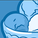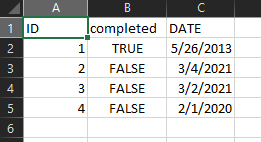Announcements
Applications are open for the 2024 Qlik Luminary Program. Apply by December 15 here.
cancel
Showing results for
Did you mean:Contributor II

## Count of day between date range

Hello Experts,

I am trying to make 3 separate KPI's in which I want to see the count of data for

1. Last 90 days
2. 179 day -91 day
3. 181 days and greater

I have a date column MM/DD/YYYY and an ID column

Thank you

2 Solutions

Accepted SolutionsSpecialist

Replace [date column] with the name of your actual date column and try this:

1.  count({\$<[date column]={">\$(=Date(Today()-90))"}>} ID)

2.  count({\$<[date column]={">=\$(=Date(Today()-179))<=\$(=Date(Today()-91))"}>} ID)

3.  count({\$<[date column]={"<=\$(=Date(Today()-180))"}>} ID)Specialist

count({\$<[DATE]={">=(Today()-179)<=(Today()-91)"}>} ID)

With completed option:

count({\$<[DATE]={">=(Today()-179)<=(Today()-91)"},[completed]={'TRUE'}>} ID)

8 RepliesSpecialist

Replace [date column] with the name of your actual date column and try this:

1.  count({\$<[date column]={">\$(=Date(Today()-90))"}>} ID)

2.  count({\$<[date column]={">=\$(=Date(Today()-179))<=\$(=Date(Today()-91))"}>} ID)

3.  count({\$<[date column]={"<=\$(=Date(Today()-180))"}>} ID)Contributor II
Author

Thank you GaryGiles for the quick solution.

The above expressions are giving me a 0.Specialist

Can you the expressions that you used?  Is your date field a true date?Contributor II
Author

So I am getting the date in "2015-10-18 00:00:00" from the database which I am converting to

" Date(Floor("DATE"), 'MM-DD-YYYY')    AS "DATE" in the load editor.Specialist

I meant post the expression(s) that are returning 0.Contributor II
Author

Also, if I use the below expression is giving me the correct count.

Last 90 days:    Count({\$<[DATE] = {"=[DATE] > (Today()-90)"}>} [ID])

181 day and greater:  Count({\$<[DATE] = {"=[DATE] < (Today()-180)"}>} [ID])

I am not sure how to write it for 179 day -91 days.

Also, how can I add a where clause to the above expression.

Eg.  count of data for the last 90 day where complete is trueContributor II
Author

All the 3 expressions you mention are returning 0.Specialist

count({\$<[DATE]={">=(Today()-179)<=(Today()-91)"}>} ID)

With completed option:

count({\$<[DATE]={">=(Today()-179)<=(Today()-91)"},[completed]={'TRUE'}>} ID)Tags
Community Browser